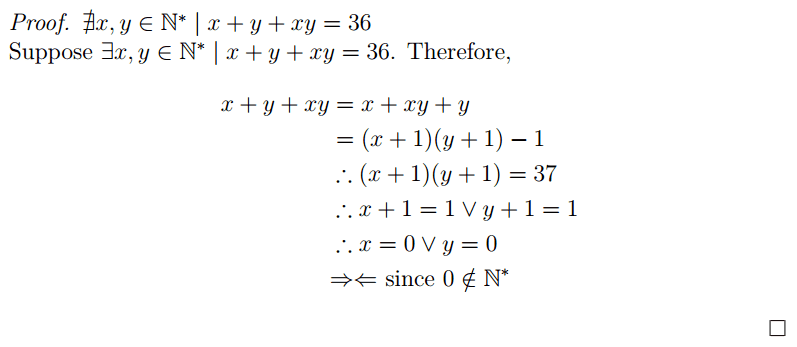# Small number theory problem

mafagafo

## Homework Statement

This is a problem I had as a margin note in an old notebook that I will recycle. I want write it using LaTeX. Problem is that I also want to write it using "proper" math notation instead of English words.

Firstly, I got this:
$$\textrm{Proof that }\nexists x, y \in \mathbb{N}^* | x + y + xy = 36$$

Which seems to be correct. (Should 'x, y' be between parentheses?)

None.

## The Attempt at a Solution

\begin{gather} \begin{aligned} x + y + xy &= x + xy + y \\ &= (x + 1) (y + 1) - 1 \\ &\therefore (x + 1) (y + 1) = 37 \\ &\therefore x = 0 \lor y = 0 \notag \end{aligned} \end{gather}

Now the question: how to finish this? Obviously x = 0 or y = 0 is false as neither x nor y can be 0 as the set of all N but 0 does not include 0. How do I write this?

Homework Helper
That's pretty good but you need to say a bit more about what you are doing. You should, for example, write
that since 37 is a prime number either x+ 1= 1 and y+ 1= 37 or x+ 1= 37 and y+ 1= 1.

Then look at the two cases: if x+ 1= 1 then x= 0. But that is a contradiction because x must be a positive integer
If y+ 1= 1 then y= 0. But that is a contradiction because y must be a positive integer.

mafagafo
All right, I will write more! But first you need to teach me how (or point me a not too long book / guide / tutorial).

Which symbols do I use? I remember some '#' for "absurd" or "impossible" on paper, but I have no idea on how to do it here. Neither if that was correct.

Mentor

## Homework Statement

This is a problem I had as a margin note in an old notebook that I will recycle. I want write it using LaTeX. Problem is that I also want to write it using "proper" math notation instead of English words.

Firstly, I got this:
$$\textrm{Proof that }\nexists x, y \in \mathbb{N}^* | x + y + xy = 36$$

Which seems to be correct. (Should 'x, y' be between parentheses?)

None.

## The Attempt at a Solution

\begin{gather} \begin{aligned} x + y + xy &= x + xy + y \\ &= (x + 1) (y + 1) - 1 \\ &\therefore (x + 1) (y + 1) = 37 \\ &\therefore x = 0 \lor y = 0 \notag \end{aligned} \end{gather}
What I would do is start by assuming that x + xy + y = 36.
Then x + xy + y + 1 - 1 = 36
##\Rightarrow## (x + 1)(y + 1) = 37
Since 37 is prime, its only factors are 1 and 37, so there's your contradiction.

As far as I can tell, there's no LaTeX symbol that specifically denotes "contradiction." Some people use # for this purpose. A symbol I've seen for a long time is two arrows with their heads touching, like this: ##\Rightarrow\Leftarrow##.

mafagafo said:
Now the question: how to finish this? Obviously x = 0 or y = 0 is false as neither x nor y can be 0 as the set of all N but 0 does not include 0. How do I write this?

mafagafo
What I would do is start by assuming that x + xy + y = 36.
Then x + xy + y + 1 - 1 = 36
##\Rightarrow## (x + 1)(y + 1) = 37
Since 37 is prime, its only factors are 1 and 37, so there's your contradiction.

As far as I can tell, there's no LaTeX symbol that specifically denotes "contradiction." Some people use # for this purpose. A symbol I've seen for a long time is two arrows with their heads touching, like this: ##\Rightarrow\Leftarrow##.
Were you going to comment something else then changed your mind?

It follows that x = 0 or y = 0 because either x + 1 = 1 or y + 1 = 1.

Will look up that symbol you wrote.

Mentor
Were you going to comment something else then changed your mind?
At first I didn't understand how you arrived at x = 0 or y = 0, but saw you had explained it following your proof, so I removed my question.
mafagafo said:
It follows that x = 0 or y = 0 because either x + 1 = 1 or y + 1 = 1.

Will look up that symbol you wrote.
It's two symbols: # #\Rightarrow\Leftarrow # #
The spaces between the # characters is to keep the above from rendering...

mafagafo
I know how to make it, I just want to look it up in a book or Wikipedia to get some usage examples.

In fact, as you may know, unless you use an image with an obscure filename*, I just need to quote you in order to see your $\LaTeX$ code.

* Even if it was an image, there are still ways to try to get LaTeX from it.

Mentor
mafagafo
The best thing I got from this thread. Thanks.
It makes me sad that Q.E.A. is not widely used (what inclines me towards avoiding it).
Anyway, this is a picture of what I rendered. Suggestions wanted.As Mark somehow suggested, I added one not-so-obvious extra step.